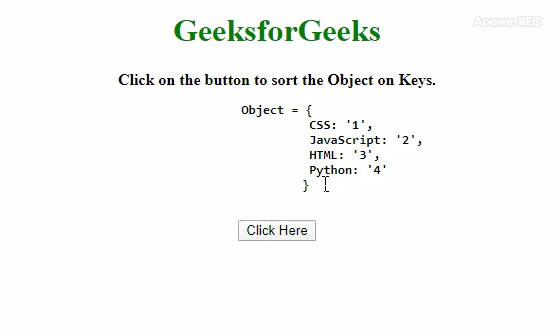# How to Sort/Order keys in JavaScript objects ?

Given an object and the task is to sort the JavaScript Object on the basis of keys. Here are a few of the most used techniques discussed with the help of JavaScript.

Approach 1: By using .sort() method to sort the keys according to the conditions specified in the function and get the sorted keys in the array. To copy the whole object to that temporary variable in the order of keys in a key array(in sorted fashion) and delete the original object will make a temporary variable. After that, we just need to copy the temporary object to the original object and return it.

• Example 1: This example implements the above approach.

 ` ` `<``html``> ` ` `  `<``head``> ` `    ``<``title``> ` `        ``How to Sort/Order keys ` `        ``in JavaScript objects ? ` `    `` ` `     `  `    ``<``style``> ` `        ``body { ` `            ``text-align: center; ` `        ``} ` `        ``h1 { ` `            ``color: green; ` `        ``} ` `        ``#geeks { ` `            ``font-size: 20px;  ` `            ``font-weight: bold; ` `        ``} ` `    `` ` ` ` ` `  `<``body``> ` `    ``<``h1``>GeeksforGeeks ` `     `  `    ``<``b``> ` `        ``Click on the button to sort  ` `        ``the Object on Keys. ` `        ``<``br``> ` `        ``<``pre``>Object = { ` `                    ``CSS: '1', ` `                    ``JavaScript: '2', ` `                    ``HTML: '3', ` `                    ``Python: '4' ` `                    ``} ` `        `` ` `    `` ` `     `  `    ``<``button` `onclick``=``"gfg_Run()"``> ` `        ``Click Here ` `    `` ` `     `  `    ``<``p` `id``=``"geeks"``> ` `     `  `    ``<``script``> ` `        ``var el_down = document.getElementById("geeks"); ` `         `  `        ``var GFG_Object = { ` `            ``CSS: '1', ` `            ``JavaScript: '2', ` `            ``HTML: '3', ` `            ``Python: '4' ` `        ``}; ` ` `  `        ``// Sorted keys are obtained in 'key' array ` `        ``function sortKeys(obj_1) { ` `            ``var key = Object.keys(obj_1) ` `            ``.sort(function order(key1, key2) { ` `                ``if (key1 < ``key2``) return -1; ` `                ``else if (key1 > key2) return +1; ` `                ``else return 0; ` `            ``});  ` `             `  `            ``// Taking the object in 'temp' object ` `            ``// and deleting the original object. ` `            ``var temp = {}; ` `             `  `            ``for (var i = 0; i < ``key.length``; i++) { ` `                ``temp[key[i]] = obj_1[key[i]]; ` `                ``delete obj_1[key[i]]; ` `            ``}  ` ` `  `            ``// Copying the object from 'temp' to  ` `            ``// 'original object'. ` `            ``for (var ``i` `= ``0``; i < key.length; i++) { ` `                ``obj_1[key[i]] = temp[key[i]]; ` `            ``}  ` `            ``return obj_1; ` `        ``} ` ` `  `        ``function gfg_Run() { ` `            ``el_down.innerHTML` `= ``JSON` `            ``.stringify(sortKeys(GFG_Object)); ` `        ``} ` `    `` ` ` ` ` `  ` `

• Output:• Approach 2: In this approach .sort() method sort the keys alphabetically then we have to use .reduce() method on these sorted keys. Then call an anonymous function inside reduce() method with 2 variables(emptyObject and key). Now one by one put the key and value pair in the emptyObject and return it.

• Example 2: This example implements the above approach.

 ` ` `<``html``> ` ` `  `<``head``> ` `    ``<``title``> ` `        ``How to Sort/Order keys ` `        ``in JavaScript objects ? ` `    `` ` `     `  `    ``<``style``> ` `        ``body { ` `            ``text-align: center; ` `        ``} ` `        ``h1 { ` `            ``color: green; ` `        ``} ` `        ``#geeks { ` `            ``font-size: 20px;  ` `            ``font-weight: bold; ` `        ``} ` `    `` ` ` ` ` `  `<``body``> ` `    ``<``h1``>GeeksforGeeks ` `     `  `    ``<``b``> ` `        ``Click on the button to sort  ` `        ``the Object on Keys.<``br``> ` `        ``<``pre``>Object = { ` `                ``CSS: '1', ` `                ``JavaScript: '2', ` `                ``HTML: '3', ` `                ``Python: '4' ` `            ``} ` `        `` ` `    `` ` `     `  `    ``<``button` `onclick``=``"gfg_Run()"``> ` `        ``Click Here ` `    `` ` `     `  `    ``<``p` `id``=``"geeks"``> ` `     `  `    ``<``script``> ` `        ``var el_down = document.getElementById("geeks"); ` `     `  `        ``var GFG_Object = { ` `            ``CSS: '1', ` `            ``JavaScript: '2', ` `            ``HTML: '3', ` `            ``Python: '4' ` `        ``}; ` ` `  `        ``function gfg_Run() { ` `             `  `            ``// Getting the keys of JavaScript Object. ` `            ``Modified_Object = Object.keys(GFG_Object)  ` `             `  `            ``// Sort and calling a method on ` `            ``// keys on sorted fashion. ` `            ``.sort().reduce(function(Obj, key) {  ` `                     `  `                ``// Adding the key-value pair to the ` `                ``// new object in sorted keys manner ` `                ``Obj[key] = GFG_Object[key];  ` `                ``return Obj;  ` `            ``}, {}); ` `            ``el_down.innerHTML =  ` `                    ``JSON.stringify(Modified_Object); ` `        ``} ` `    `` ` ` ` ` `  ` `

• Output:Whether you're preparing for your first job interview or aiming to upskill in this ever-evolving tech landscape, GeeksforGeeks Courses are your key to success. We provide top-quality content at affordable prices, all geared towards accelerating your growth in a time-bound manner. Join the millions we've already empowered, and we're here to do the same for you. Don't miss out - check it out now!

Previous
Next